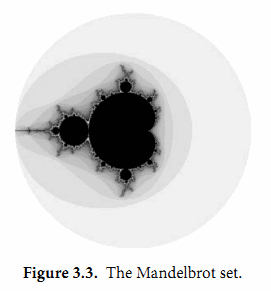## 3.3. 复数

Go语言提供了两种精度的复数类型：complex64和complex128，分别对应float32和float64两种浮点数精度。内置的complex函数用于构建复数，内建的real和imag函数分别返回复数的实部和虚部：

var x complex128 = complex(1, 2) // 1+2i
var y complex128 = complex(3, 4) // 3+4i
fmt.Println(x*y)                 // "(-5+10i)"
fmt.Println(real(x*y))           // "-5"
fmt.Println(imag(x*y))           // "10"


fmt.Println(1i * 1i) // "(-1+0i)", i^2 = -1


x := 1 + 2i
y := 3 + 4i


math/cmplx包提供了复数处理的许多函数，例如求复数的平方根函数和求幂函数。

fmt.Println(cmplx.Sqrt(-1)) // "(0+1i)"


gopl.io/ch3/mandelbrot

// Mandelbrot emits a PNG image of the Mandelbrot fractal.
package main

import (
"image"
"image/color"
"image/png"
"math/cmplx"
"os"
)

func main() {
const (
xmin, ymin, xmax, ymax = -2, -2, +2, +2
width, height          = 1024, 1024
)

img := image.NewRGBA(image.Rect(0, 0, width, height))
for py := 0; py < height; py++ {
y := float64(py)/height*(ymax-ymin) + ymin
for px := 0; px < width; px++ {
x := float64(px)/width*(xmax-xmin) + xmin
z := complex(x, y)
// Image point (px, py) represents complex value z.
img.Set(px, py, mandelbrot(z))
}
}
png.Encode(os.Stdout, img) // NOTE: ignoring errors
}

func mandelbrot(z complex128) color.Color {
const iterations = 200
const contrast = 15

var v complex128
for n := uint8(0); n < iterations; n++ {
v = v*v + z
if cmplx.Abs(v) > 2 {
return color.Gray{255 - contrast*n}
}
}
return color.Black
}